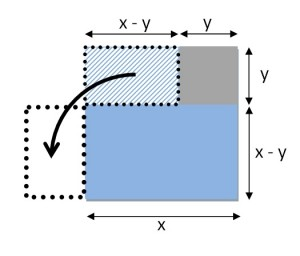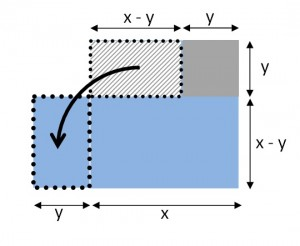It Really is the Difference…

…of two squares.

Suppose we start with a square with side x and remove a smaller square with side y.

The remaining area (shown in blue) is literally “the difference of two squares”.We can find its area by subtracting the little square from the big one to get:

Area = x2 – y2

But we can also find the area another way.  Let’s cut things up and move them around.As you can see, the area we were looking for is also the area of a rectangle.  The length is (x + y) and the width is (x – y).  That gives us a new formula for this area:

Area = (x + y)(x – y)

But we are talking about the same area both times.  So what we have now is a geometric reason to believe that the difference of two squares can be factored:

x2 – y2 = (x + y)(x – y)

How important is this?  Hmm.  Within the context of high school math (and SAT math), it is apparently one of the most important factoring facts that there is.  In physics class, I can only think of one setting where we used this. (We were deriving a rule about velocities before and after an elastic collision, in case you were wondering.)

The math we teach should either be useful or interesting.  To me, the difference of two squares is more interesting when we use pictures.  And the idea of carving up the same area two different ways to see what you learn can be very powerful and lead to unquestionably important results.  More about this in the next post.